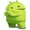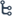பாடசாலை வலைதளத்தின் New Android App ஐ Download செய்து பயன்படுத்தவும்! - https://play.google.com/store/apps/details?id=com.padasalai.padasalai

••## CBSE Class 6 Maths - New Syllabus 2020 - 2021 DownloadCBSE Class 6 Maths Syllabus – 2020-2021 (Page 1)

 Chapter Topics Topics Chapter 1: Knowing Our Numbers 1.1 Introduction1.2 Comparing Numbers1.3 Large Numbers in Practice1.4 Using Brackets1.5 Roman Numerals Chapter 2: Whole Numbers 2.1 Introduction2.2 Whole Numbers2.3 The Number Line2.4 Properties Of Whole Numbers2.5 Patterns in Whole Numbers Chapter 3: Playing With Numbers 3.1 Introduction3.2 Factors and Multiples3.3 Prime and Composite Numbers3.4 Test For Divisibility Of Numbers3.5 Common Factors and Common Multiples3.6 Some More Divisibility Rules3.7 Prime Factorisation3.8 Highest Common Factor3.9 Lowest Common Multiple3.10 Some Problems on HCF and LCM

CBSE Class 6 Maths Syllabus – 2020-2021 (Page 2)

 Chapter Topics Topics Chapter 4: Basic Geometrical Ideas 4.1 Introduction4.2 Points4.3 A Line Segment4.4 A line4.5 Intersecting Lines4.6 Parallel Lines4.7 Ray4.8 Curves4.9 Polygons4.10 Angles4.11 Triangles4.12 Quadrilaterals4.13 Circles Chapter 5: Understanding Elementary Shapes 5.1 Introduction5.2 Measuring Line Segments5.3 Angles-’Right’ and ‘Straight’5.4 Angles- ‘Acute’, ‘Obtuse’ and ‘Reflex’5.5 Measuring Angles5.6 Perpendicular Lines5.7 Classification of Triangles5.8 Quadrilaterals5.9 Polygons5.10 Three Dimensional Shapes Chapter 6: Integers 6.1 Introduction6.2 Integers6.3 Addition of Integers6.4 Subtraction of Integers with the help of a NumberLine

CBSE Class 6 Maths Syllabus – 2020-2021 (Page 3)

 Chapter Topics Topics Chapter 7: Fractions 7.1 Introduction7.2 A Fraction7.3 Fraction on the Number Line7.4 Proper Fractions7.5 Improper and Mixed Fractions7.6 Equivalent Fractions7.7 Simplest Form of a Fraction7.8 Like Fractions7.9 Comparing Fractions7.10 Addition and Subtraction of Fractions Chapter 8: Decimals 8.1 Introduction8.2 Tenths8.3 Hundredths8.4 Comparing Decimals8.5 Using Decimals8.6 Addition of Numbers with Decimals8.7 Subtraction of Decimals Chapter 9: Data Handling 9.1 Introduction9.2 Recording Data9.3 Organisation of Data9.4 Pictograph9.5 Interpretation of a Pictograph9.6 Drawing a Pictograph9.7 A Bar Graph Chapter 10: Mensuration 10.1 Introduction10.2 Perimeter10.3 Area

CBSE Class 6 Maths Syllabus – 2020-2021 (Page 4)

 Chapter Topics Topics Chapter 11: Algebra 11.1 Introduction11.2 Matchstick Patterns11.3 The Idea Of A Variable11.4 More Matchstick Patterns11.5 More Examples of Variables11.6 Use Of Variables in Common Rules11.7 Expressions with Variables11.8 Using Expressions Practically11.9 What is an Equation?11.10 Solution of an Equation Chapter 12: Ratio and Proportion 12.1 Introduction12.2 Ratio12.3 Proportion12.4 Unitary Method Chapter 13: Symmetry 13.1 Introduction13.2 Making Symmetric Figures: Ink-blot Devils13.3 Figures With Two Lines of Symmetry13.4 Figures with Multiple Lines of Symmetry13.5 Reflection and Symmetry Chapter 14: Practical Geometry 14.1 Introduction14.2 The Circle14.3 A Line Segment14.4 Perpendiculars14.5 Angles